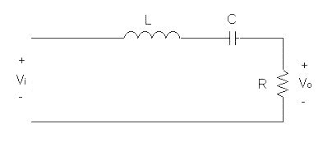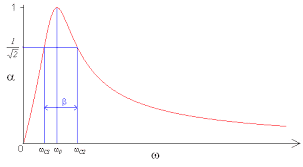# Series bandpass filter graph

so_gr_lo
Homework Statement:
I am plotting Vout/Vin against frequency for a RLC series bandpass filter, where Vout is across the resistor. The resulting graph has max Vout/Vin of about 1.5, the max is supposed to be 1 in a bandpass filter. The Vout/Vin values are experimental, could a max Vout/Vin of 1.5 occur in an experiment? And what could be the reason for this?
Relevant Equations:
Vin/Vout = R / (R+wL -1/wc) , where w = omega
this is the circuitthis is the theoretical graphHomework Helper
Hi,

Your relevant equation is an equation with complex quantities on both sides.
(I think you switched in and out subscripts ?)

What you measure is most likely the real ratio of two amplitudes. As plotted in the theoretical graph.
For the complex impedance of the RLC series we have $$Z^2 = R^2 + \left (\omega L - {1\over \omega C}\right )^2$$ with a minimum ##Z=R## (and ##V_{out}/V_{in}=1## ).

A measured ratio can deviate if the measurement equipment influences the circuit. So you have to check values of components and equipment.

##\ ##

so_gr_lo
So what equation could you use to plot theoretical values of Vout / Vin ?